Home > CC1 > Chapter 8 > Lesson 8.3.3 > Problem8-119

8-119.
1. Evaluate each of the following expressions for x = 10. Leave your answers as fractions. Show your work. Homework Help ✎

1.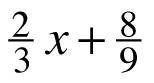2.x − 41

3. x + x +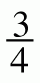x + 22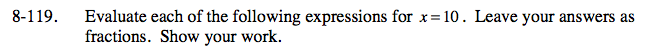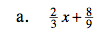Substitute 10 for the variable.

$\left ( \frac{2}{3}\cdot 10 \right )+\frac{8}{9}$

Use the order of operations:

Find the common denominator.

$7\frac{5}{9}$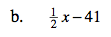Substitute 10 for the variable.

Use the order of operations to evaluate the expression below.

$\frac{10}{2} - 41$

Simplify.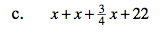Follow the same steps as parts (a) and (b).